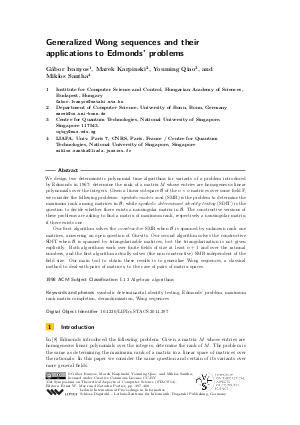Document# Generalized Wong sequences and their applications to Edmonds' problems

### Authors Gábor Ivanyos, Marek Karpinski, Youming Qiao, Miklos Santha## File

LIPIcs.STACS.2014.397.pdf
• Filesize: 0.66 MB
• 12 pages

## Cite As

Gábor Ivanyos, Marek Karpinski, Youming Qiao, and Miklos Santha. Generalized Wong sequences and their applications to Edmonds' problems. In 31st International Symposium on Theoretical Aspects of Computer Science (STACS 2014). Leibniz International Proceedings in Informatics (LIPIcs), Volume 25, pp. 397-408, Schloss Dagstuhl - Leibniz-Zentrum für Informatik (2014)
https://doi.org/10.4230/LIPIcs.STACS.2014.397

## Abstract

We design two deterministic polynomial time algorithms for variants of a problem introduced by Edmonds in 1967: determine the rank of a matrix M whose entries are homogeneous linear polynomials over the integers. Given a linear subspace B of the nxn matrices over some field F, we consider the following problems: symbolic matrix rank (SMR) is the problem to determine the maximum rank among matrices in B, while symbolic determinant identity testing (SDIT) is the question to decide whether there exists a nonsingular matrix in B. The constructive versions of these problems are asking to find a matrix of maximum rank, respectively a nonsingular matrix, if there exists one. Our first algorithm solves the constructive SMR when B is spanned by unknown rank one matrices, answering an open question of Gurvits. Our second algorithm solves the constructive SDIT when B is spanned by triangularizable matrices, but the triangularization is not given explicitly. Both algorithms work over finite fields of size at least n+1 and over the rational numbers, and the first algorithm actually solves (the non-constructive) SMR independent of the field size. Our main tool to obtain these results is to generalize Wong sequences, a classical method to deal with pairs of matrices, to the case of pairs of matrix spaces.
##### Keywords
• symbolic determinantal identity testing
• Edmonds' problem
• maximum rank matrix completion
• derandomization
• Wong sequences

## Metrics

• Access Statistics
• Total Accesses (updated on a weekly basis)
0